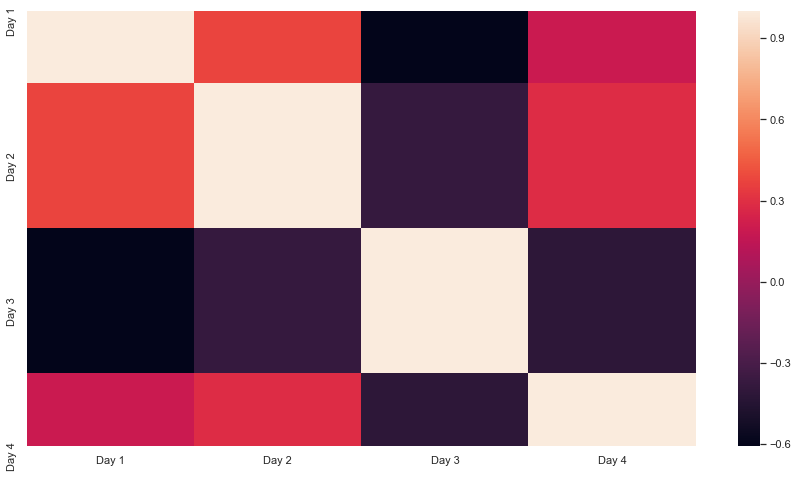# 设置 Seaborn 热图的大小

## 使用 `seaborn.set()` 函数设置 seaborn 热图大小

`set()` 函数定义了 seaborn 绘图的配置和主题。我们可以在 `rc` 参数中提及绘图的大小。

``````import pandas as pd
import matplotlib.pyplot as plt
import seaborn as sns
df = pd.DataFrame({"Day 1": [7,1,5,6,3,10,5,8],
"Day 2" : [1,2,8,4,3,9,5,2],
"Day 3" : [4,6,5,8,6,1,2,3],
"Day 4" : [5,8,9,5,1,7,8,9]})
sns.set(rc = {'figure.figsize':(15,8)})
sns.heatmap(df.corr())
``````## 使用 `matplotlib.pyplot.figure()` 函数设置 seaborn 热图大小

`figure()` 函数用于在 Python 中启动或自定义当前图形。此图中绘制了热图。可以使用函数中的 `figsize` 参数更改大小。

``````import pandas as pd
import matplotlib.pyplot as plt
import seaborn as sns
df = pd.DataFrame({"Day 1": [7,1,5,6,3,10,5,8],
"Day 2" : [1,2,8,4,3,9,5,2],
"Day 3" : [4,6,5,8,6,1,2,3],
"Day 4" : [5,8,9,5,1,7,8,9]})
plt.figure(figsize = (15,8))
sns.heatmap(df.corr())
``````## 使用 `matplotlib.pyplot.gcf()` 函数设置 seaborn 图的大小

`gcf()` 函数返回图形的视图实例对象。可以使用 `set_size_inches()` 方法更改此对象的大小。通过这种方式，我们可以在这个对象上设置热图图的大小。

``````import pandas as pd
import matplotlib.pyplot as plt
import seaborn as sns
df = pd.DataFrame({"Day 1": [7,1,5,6,3,10,5,8],
"Day 2" : [1,2,8,4,3,9,5,2],
"Day 3" : [4,6,5,8,6,1,2,3],
"Day 4" : [5,8,9,5,1,7,8,9]})
sns.heatmap(df.corr())
plt.gcf().set_size_inches(15, 8)
``````